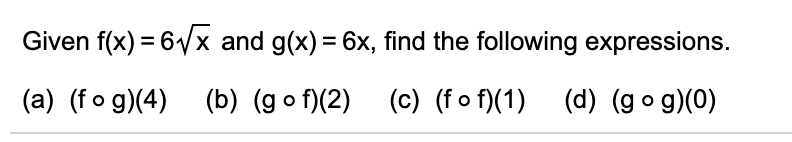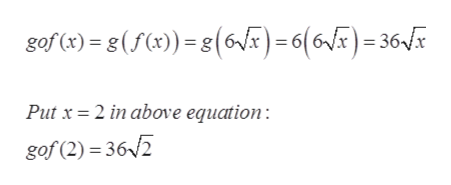# Given f(x) = 6Vx and g(x) = 6x, find the following expressions.(a) (fo g)(4) (b) (go f)(2) (c) (f o f)(1) (d) (go g)(0)

Question
7 viewshelp_outlineImage TranscriptioncloseGiven f(x) = 6Vx and g(x) = 6x, find the following expressions. (a) (fo g)(4) (b) (go f)(2) (c) (f o f)(1) (d) (go g)(0) fullscreen
check_circle

Step 1

Given:

Step 2

The fog(x) of the given functions is as follows:help_outlineImage Transcriptionclosefog(x) = f (g(x))= f(6x) = 6/6x Put x = 4 in above equation: fog(4) = 6,/6(4) = 6/24 = 12/6 fullscreen
Step 3

The gof(x) of the given functi...help_outlineImage Transcriptionclosegof (x) = g(f(x)) = g(VF)=6(6/x)=36x Put x = 2 in above equation : gof (2) = 36/2 fullscreen

### Want to see the full answer?

See Solution

#### Want to see this answer and more?

Solutions are written by subject experts who are available 24/7. Questions are typically answered within 1 hour.*

See Solution
*Response times may vary by subject and question.
Tagged in

### Algebra